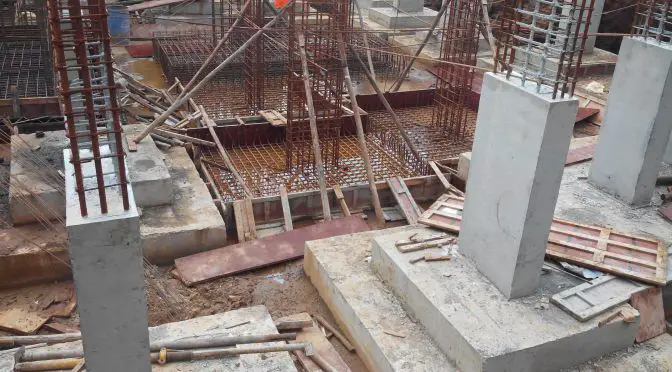# On the Bearing Capacity of Shallow FoundationsBearing capacity is the maximum load a soil profile can withstand before undergoing excessive deformation and shear failure. It is the most popular and perhaps the most important information needed for the design of shallow foundations. The allowable bearing capacity of soil is used for the proper sizing of shallow foundations so that the load from the superstructure will not exceed the strength of the soil or lead to an excessive settlement.

If a load is applied gradually to a foundation, the settlement will increase. At a certain point when the load equals the bearing capacity of the soil, sudden failure of the soil supporting the foundation will take place. This sudden failure in which the failure surface will extend into the ground is known as ‘general shear failure‘.

When the soil supporting the foundation is of sand or clay soil of medium compaction, the failure surface of the soil will gradually extend outward from the foundation. When the applied load reaches the bearing capacity of the soil, the foundation movement will be accompanied by sudden jerks, and considerable movement will be required before the failure surface extends into the ground. This is generally referred to as ‘local shear failure‘, and the peak value of the load is not realised in this type of failure.

If the foundation is founded on loose soil, the failure surface will not extend into the ground surface. The load-settlement plot of this interaction will be steep and practically linear. Such failure is referred to as ‘punching shear failure‘.

The methods of determining the ultimate bearing capacity of soils are;

• General shear failure theory of Terzaghi
• Theoretical solutions presented by Meyerhof, Hansen, and Vesic
• Correlations from in-situ tests such as PLT, SPT, and CPT

Information needed for the evaluation of the bearing capacity of soil is obtained from site investigation. Laboratory study of undisturbed samples or in-situ soil tests can be done in order to obtain the shear strength parameters needed for the evaluation of the bearing capacity. Several correlations exist for relating in-situ soil properties from cone penetration test to bearing capacity of the soil.

Soil investigation is therefore one of the most important activities that must be carried out before the commencement of any construction project. In the soil test report, the geotechnical engineer is expected to state the strength of the soil at different layers, and ultimately recommend a suitable foundation. Some of the parameters used in describing the strength of a soil formation for the purposes of estimating the soil bearing capacity are the cohesion and the angle of internal friction of the soil.

In this article, we are going to present an example of how to determine the bearing capacity of soil using the general bearing capacity equation.

## Background to the bearing capacity of shallow foundations

Terzaghi in 1943 extended the plastic failure theory of Prandtl to evaluate the bearing capacity for shallow strip footings. After the development of Terzaghi’s bearing capacity equation, several scholars such as Meyerhof (1951 and 1963), Vesic (1973), Hansen (1970), etc worked on this area and refined the solution to what is known as the general bearing capacity equation. This modification allowed for depth factor, shape factor, and inclination factors.

The modified general ultimate bearing capacity equation can be written as;

qu = c’FcsFcdFciNc + qFqsFqdFqiNq + 0.5FγsFγdFγiγBNγ

Where;
Fcs, Fqs, Fγs are shape factors which account for the shearing resistance developed along the surface in soil above the base of the footing
Fcd, Fqd, Fγd are depth factors to determine the bearing capacity of rectangular and circular footings
Fci, Fqi, Fγi are inclination factors to determine the bearing capacity of a footing on which the direction of load application is inclined at a certain angle to the vertical

## Solved Exampleon the determination of bearing capacity

Let us determine the bearing capacity of a simple pad foundation with the following data;

Depth of foundation Df = 0.9 m
Width of foundation B = 1.0 m
Effective cohesion of soil c’ = 12 kN/m2
Angle of internal friction φ’ = 27°
Unit weight of soil = 18.5 kN/m3

The water table is about 9 m below the surface

From table, we can determine the bearing capacity factors;

Angle of internal friction φ’ = 27°
Nc = 23.94; Nq = 13.20; Nγ = 14.47

Fcs = 1 + (B/L)(Nq /Nc) = 1 + (1.0/1.0)(13.2/23.94) = 1.551
Fqs = 1 + (B/L)tanφ’  = 1 + (1.0/1.0)tan 27 = 1.509
Fγs = 1 + 0.4(B/L)  = 1 + 0.4(1.0/1.0) = 1.4

Fcd = 1 + 0.4(Df/B)  = 1 + 0.4(0.9/1.0) = 1.36
Fqd = 1 + 2tanφ'(1 – sin φ’)2(Df/B)  = 1 + 2tan27(1 – sin 27)2 (0.9/1.0) = 1.273
Fγd = 1.0

Since we are assuming vertical loads, take Fci = Fqi = Fγi = 1.0

q = (18.5 kN/m3 × 0.9 m) = 16.65 kN/m2

qu = c’FcsFcdFciNc + qFqsFqdFqiNq + 0.5FγsFγdFγiγBNγ
qu = (12 × 23.94 × 1.551 × 1.36 × 1.0) + (16.65 × 1.509 × 1.273 × 1.0 × 13.20) + (0.5 × 1.4 × 1.0 × 1.0 × 18.5 × 1.0 × 14.47) = 1215.55 kN/m2

Using a factor of safety (FOS) of 3.0
qallowable = qu /FOS = 1215.55/3.0 = 405.183 kN/m2

So with this, the allowable bearing capacity of the soil can be stated as 405 kN/m2

1.Getalem Worku

I like this site very much!!

•Ubani Obinna

Thank you very much, and keep visiting

2.syed kashif

brother, you dint multiplied by unit wt of soil in this equation i.e (0.5FYs*Fyd*Fyi*YB*Ny) = (0.5*1.4*1*1*1*18.5*14.47) = 187.386 kn/m2

please correct me, if am wrong

•Ubani Obinna

The correction has been effected. Thanks for pointing that out.

3.Amoo olaoluwa

Thanks for this

4.Samer

How can we calculate the bearing capacity of an isolated footing resting on multi layered soil with thin layers????